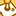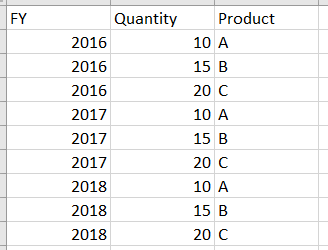# New to Qlik Sense

If you’re new to Qlik Sense, start with this Discussion Board and get up-to-speed quickly.

Announcements
cancel
Showing results for
Did you mean:Contributor III

## Sum formula Pivot table

Hello guys,

can someone help me?

• I have a pivot table with dimension "Product"
• in the column I want to see the Quantity, but only from the highest selected year. (so if i select in a filter FY 2016,2017 and 2018) I only want to see the sum of the Quantity of 2018, and if I would select FY 2016 and 2017 I only want to see se sum of the Quantity of 2017, and so on...

if i insert "sum(Quantity)" i get always the sum of all selected FYs. Any idea how that could work?

Many thanks for any help!

Best Markus1 Solution

Accepted SolutionsMVP

I think you mean this

Sum({<year = {"\$(=max(year))"}>} Quantity)

Sum({<FY = {"\$(=max(FY))"}>} Quantity)

6 RepliesPartner

maybe this:

sum( {< year = {"=max(year)"} >} Quantity )

I hope it helps.Contributor III
AuthorPartner

did you try with this below ?

sum( {< FY = {"=max(FY)"} >} Quantity )

FY field should be an external filter and not a pivot dimension.Contributor III
Author

yes i changed year to FYMVP

I think you mean this

Sum({<year = {"\$(=max(year))"}>} Quantity)

Sum({<FY = {"\$(=max(FY))"}>} Quantity)Contributor III
Author

Thank you Sunny and Andrea!!